# 算法-动态规划 Dynamic Programming–从菜鸟到老鸟

## 动态规划算法的核心A * “1+1+1+1+1+1+1+1 =？” *

A : “上面等式的值是多少”
B : 计算 “8!”

A 在上面等式的左边写上 “1+” *
A : “此时等式的值为多少”
B : *quickly
“9!”
A : “你怎么这么快就知道答案了”
A : “只要在8的基础上加1就行了”
A : “所以你不用重新计算因为你记住了第一个等式的值为8!动态规划算法也可以说是 ‘记住求过的解来节省时间'”

## 动态规划算法的两种形式

Fibonacci (n) = 1;   n = 0

Fibonacci (n) = 1;   n = 1

Fibonacci (n) = Fibonacci(n-1) + Fibonacci(n-2)


public int fib(int n) {
if (n <= 0) {
return 0;
}
if (n == 1) {
return 1;
}
return fib(n - 1) + fib(n - 2);
}
//输入6
//输出：8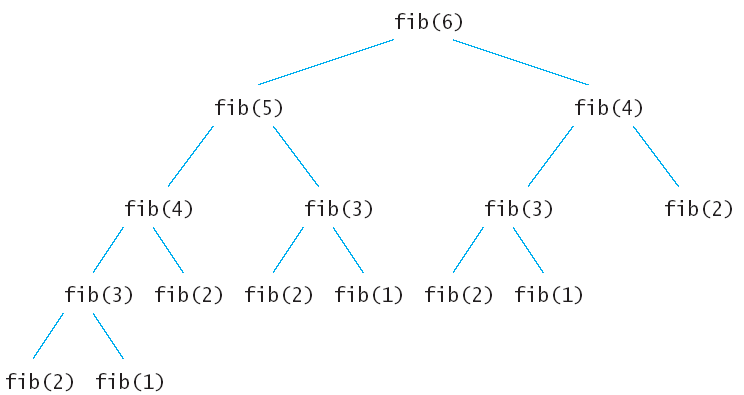### ①自顶向下的备忘录法

public static int Fibonacci(int n) {
if (n <= 0) {
return n;
}
int[] Memo = new int[n + 1];
for (int i = 0; i <= n; i++) {
Memo[i] = -1;
}
return fib(n, Memo);
}

public static int fib(int n, int[] Memo) {
if (Memo[n] != -1) {
return Memo[n];
}
//如果已经求出了fib（n）的值直接返回，否则将求出的值保存在Memo备忘录中。
if (n <= 2) {
Memo[n] = 1;
} else {
Memo[n] = fib(n - 1, Memo) + fib(n - 2, Memo);
}

return Memo[n];
}


### ②自底向上的动态规划

public static int fib(int n) {
if (n <= 0) {
return n;
}
int[] Memo = new int[n + 1];
Memo = 0;
Memo = 1;
for (int i = 2; i <= n; i++) {
Memo[i] = Memo[i - 1] + Memo[i - 2];
}
return Memo[n];
}


public static int fib(int n) {
if (n <= 1) {
return n;
}

int Memo_i_2 = 0;
int Memo_i_1 = 1;
int Memo_i = 1;
for (int i = 2; i <= n; i++) {
Memo_i = Memo_i_2 + Memo_i_1;
Memo_i_2 = Memo_i_1;
Memo_i_1 = Memo_i;
}
return Memo_i;
}


## 动态规划小试牛刀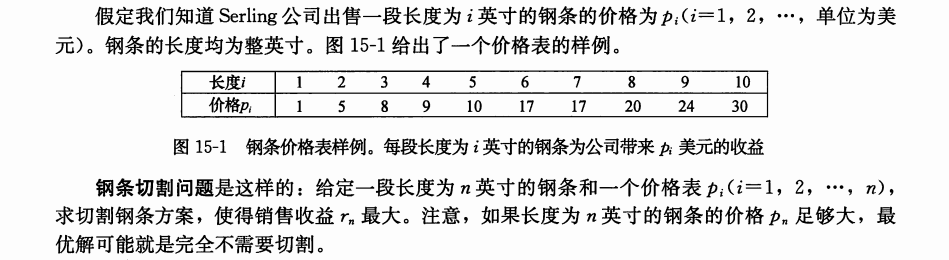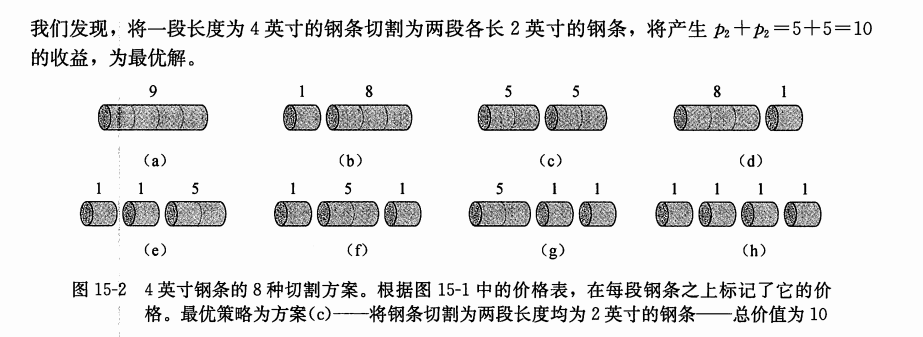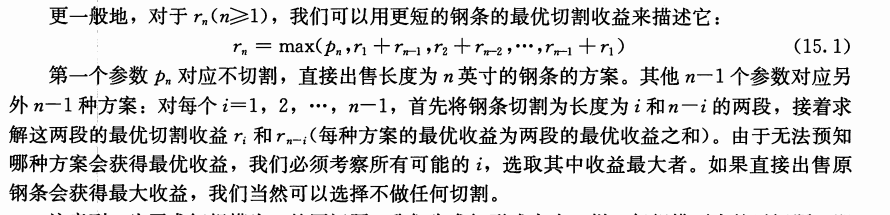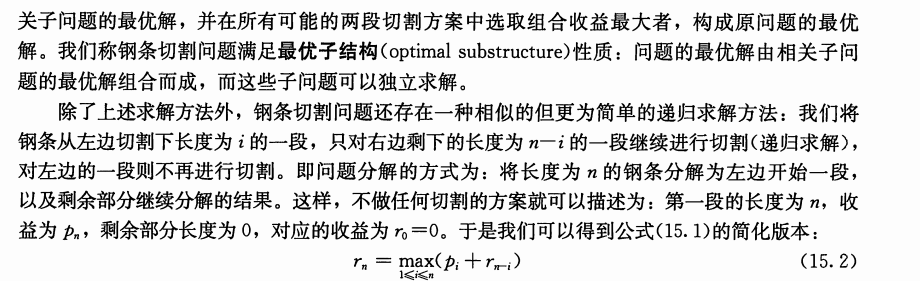### ①递归版本

public static int cut(int[] p, int n) {
if (n == 0) {
return 0;
}
int q = Integer.MIN_VALUE;
for (int i = 1; i <= n; i++) {
q = Math.max(q, p[i - 1] + cut(p, n - i));
}
return q;
}


### ②备忘录版本

public static int cutMemo(int[] p) {
int[] r = new int[p.length + 1];
for (int i = 0; i <= p.length; i++) {
r[i] = -1;
}
return cut(p, p.length, r);
}

public static int cut(int[] p, int n, int[] r) {
int q = -1;
if (r[n] >= 0) {
return r[n];
}
if (n == 0) {
q = 0;
} else {
for (int i = 1; i <= n; i++) {
q = Math.max(q, cut(p, n - i, r) + p[i - 1]);
}
}
r[n] = q;

return q;
}


### ③自底向上的动态规划

public static int buttom_up_cut(int[] p) {
int[] r = new int[p.length + 1];
for (int i = 1; i <= p.length; i++) {
int q = -1;
//①
for (int j = 1; j <= i; j++) {
q = Math.max(q, p[j - 1] + r[i - j]);
}
r[i] = q;
}
return r[p.length];
}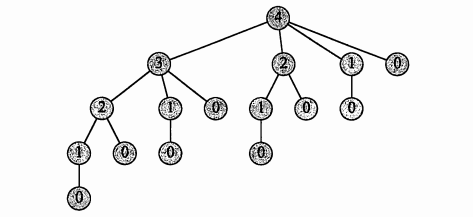## 动态规划的经典模型

### 线性模型

【例题1】在一个夜黑风高的晚上，有n（n <= 50）个小朋友在桥的这边，现在他们需要过桥，但是由于桥很窄，每次只允许不大于两人通过，他们只有一个手电筒，所以每次过桥的两个人需要把手电筒带回来，i号小朋友过桥的时间为T[i]，两个人过桥的总时间为二者中时间长者。问所有小朋友过桥的总时间最短是多少。T = minPTime * (N-2) + (totalSum-minPTime)

### 区间模型

【例题2】给定一个长度为n（n <= 1000）的字符串A，求插入最少多少个字符使得它变成一个回文串。

1、在A[j]后面添加一个字符A[i]；

2、在A[i]前面添加一个字符A[j]；

d[i][j] = min{ d[i+1][j], d[i][j-1] } + 1; (每次状态转移，区间长度增加1)

### 背包模型

【例题3】有N种物品（每种物品1件）和一个容量为V的背包。放入第 i 种物品耗费的空间是Ci，得到的价值是Wi。求解将哪些物品装入背包可使价值总和最大。f[i][v]表示前i种物品恰好放入一个容量为v的背包可以获得的最大价值。决策为第i个物品在前i-1个物品放置完毕后，是选择放还是不放，状态转移方程为：

f[i][v] = max{ f[i-1][v], f[i-1][v – Ci] +Wi }

1、最长单调子序列

2、最大M子段和

3、线性模型